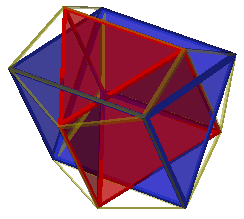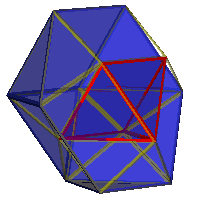Convex Segmentochora: Index of parts3p || refl ortho 3p3cup || 4pyr

 line line segment 10g decagon 3g trigon, triangle 4g square, tetragon 5g pentagon 6g hexagon 8g octagon n-g general n-gon 10ap decagonal antiprism 10p decagonal prism 2ap see tet 2cup see 3p 3ap see oct 3cup trigonal cupola, J3 half of cuboctahedron 3p trigonal prism, digonal cupola, square wedge 3pyr see tet 4ap square antiprism 4cup square cupola, J4, kind of diminished rhombicuboctahedron 4p cube, hexahedron, square prism 4pyr square pyramid, J1, trigonal wedge 5ap pentagonal antiprism, parabidiminished icosahedron 5cup pentagonal cupola, J5, kind of diminished rhombicosidodecahedron 5p pentagonal prism 5pyr pentagonal pyramid, J2, kind of diminished icosahedron 6ap hexagonal antiprism 6p hexagonal prism 8ap octagonal antiprism 8p octagonal prism, parabidiminished rhombicuboctahedron co cuboctahedron, rhombitetratetrahedron cube see 4p doe dodecahedron girco truncated cuboctahedron, great rhombicuboctahedron grid truncated icosidodecahedron, great rhombicosidodecahedron id icosidodecahedron ike icosahedron, snub tetratetrahedron J1 see 4pyr J11 gyroelongated pentagonal pyramid, kind of diminished icosahedron J19 elongated square cupola, kind of diminished rhombicuboctahedron J2 see 5pyr J27 trigonal orthobicupola, gyrated cuboctahedron J3 see 3cup J34 orthobirotunda, gyrated icosidodecahedron J37 elongated square gyrobicupola, gyrated rhombicuboctahedron J4 see 4cup J5 see 5cup J6 rotunda, half of icosidodecahedron J62 metabidiminished icosahedron J63 tridiminished icosahedron J72 gyrated rhombicosidodecahedron J73 parabigyrated rhombicosidodecahedron J74 metabigyrated rhombicosidodecahedron J75 trigyrated rhombicosidodecahedron J76 diminished rhombicosidodecahedron J77 gyrated paradiminished rhombicosidodecahedron J78 gyrated metadiminished rhombicosidodecahedron J79 bigyrated diminished rhombicosidodecahedron J80 parabidiminished rhombicosidodecahedron J81 metabidiminished rhombicosidodecahedron J82 gyrated bidiminished rhombicosidodecahedron J83 tridiminished rhombicosidodecahedron n-ap general n-gonal antiprism n-p general n-gonal prism oct octahedron, trigonal antiprism, tetratetrahedron rot see J6 sirco (small) rhombicuboctahedron snic snub cube, snub cuboctahedron snid snub dodecahedron, snub icosidodecahedron square see 4g srid (small) rhombicosidodecahedron tet tetrahedron ti truncated icosahedron tic truncated cube tid truncated dodecahedron toe truncated octahedron trig see 3g tut truncated tetrahedron

Back: To Table of Convex Segmentochora

Back: To Main Segmentochora Page

Back:  To Index